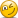# Parametric VaR

#### Jorge.Beca

##### New Member
Hi David,
I am having problems relating Absolute VaR definition with Delta-Gamma VaR definition.
For example, for a Derivative:
Absolute VaR = -u*T + volatility(S)*delta*SQRT(T)*Z = -u*T + volatility(c)*SQRT(T)*Z
Delta-Gamma VaR = Ac = delta*AS + 1/2 *Gamma*AS^2
I think I understand each formula individually:
Absolute Var = risk factor- current position
Ac = option price change to a a change in stock
...but is hard for me to relate them.

Thanks.

#### David Harper CFA FRM

##### David Harper CFA FRM
Staff member
Subscriber
Mathematically we can connect them, at the risk of missing a larger point, I think. If we need two terms of the Taylor Series (i.e., delta-gamma) then probably we are talking about an option or bond or something non-linear. (delta-normal VaR is just a special case where we only use a linear relationship with respect to the risk factor). So if we consider, say, a call option under delta-gamma VaR, then we have:
• Δc = delta*ΔS + 0.5*gamma*ΔS^2 = ΔS*[delta + 0.5*gamma*ΔS], and if we want to call it delta-gamma VaR we are just "shocking" the risk factor, ΔS, to its worst expected change with some level of confidence:
• VaR(Δc) = delta*VaR(ΔS) + 0.5*gamma*VaR(ΔS)^2 = VaR(ΔS)*[delta + 0.5*gamma*VaR(ΔS)] where VaR(ΔS) is just some large "worst case" ΔS. All we've done is be more specific than delta by adding the gamma term.
Then if we want to approximate the aVaR of an option, it could be:
• aVaR(Δc) = +Θ*Δt + [σ(X)*sqrt(Δt)*Z(α)*S(0)]*delta; and let [σ(X)*sqrt(Δt)*Z(α)*S(0)] = worst expected dollar change in stock price (aka, the risk factor) and signify this as VaR(ΔS) = [σ(X)*sqrt(Δt)*Z(α)*S(0)]. I think it's worth nothing that σ(X)*sqrt(Δt)*Z(α) simply "shocks" a per annum volatility into a worst expected stock move; e.g., if S(0) = $100.00, σ(S) = 10.0%, Z(a) = 2.33, and Δt = 2.0 years, then 10.0%*sqrt(2) = 12.1% is a 2-year volatility, 10.0%*sqrt(2)*2.33 = 32.95% is a worst expected 2-year change and 10.0%*sqrt(2)*2.33 = 32.95%*$100.00 = \$32.92 is the worst expected dollar change in the risk factor (the stock price)! So we have:
• aVaR(Δc) = +Θ*Δt + VaR(ΔS)*delta
Both have VaR(Δs) as the realized worst change in the risk factor. Both estimate our option's exposure as a delta-multiple of some worst expected change in the underlying risk factor the stock price (then one goes further by "improving on delta" by adding its gamma correction). Delta-gamma improves on the "transmission parameter" (translating the worst change in the risk factor to the worst change in the option) by adding gamma. aVaR doesn't go this far, being a typical "delta-normal" application rather than delta-gamma-normal, which is valid! Also, aVaR has a drift term; notice because its an option, my drift terms is +Θ*Δt because theta is naturally negative! So I would say:
• With respect to [delta + 0.5*gamma*VaR(ΔS)] versus VaR(ΔS)*delta, both are Taylor Series, but one is more accurate
• The drift explains the fundamental difference which I would characterize as: the delta-gamma is actually instantaneous, it does not really contemplate a long forward window (delta and gamma are unstable). I'm just thinking of this term now, so don't quote me, but I might call this a "vertical" application, as in: what's the immediate impact estimated by Taylor Series approximation. Compared to the aVaR wich, by having a drift, by definition implies a "horizontal" application going forward in time. If we weren't looking forward over a horizon, after all, the drift must be zero. It's only because we want an aVaR over a week/month/year that the drift is included. This is the difference that I think could be missed by reconciling them mathematically. I hope that's helpful, it's midnight, so i'll check my math symbols tomorrow